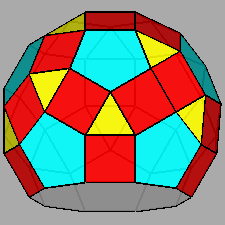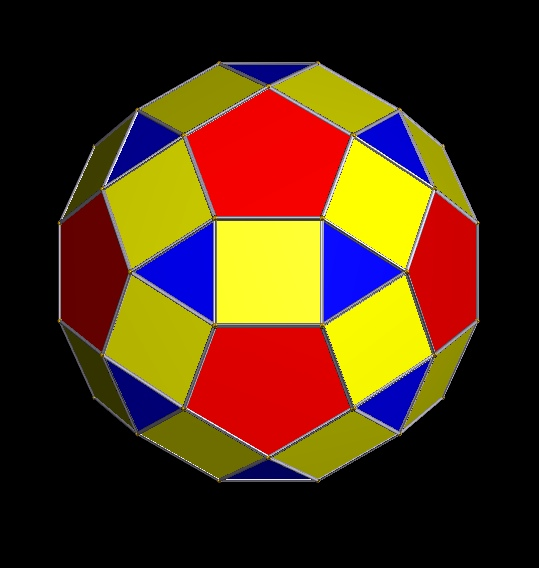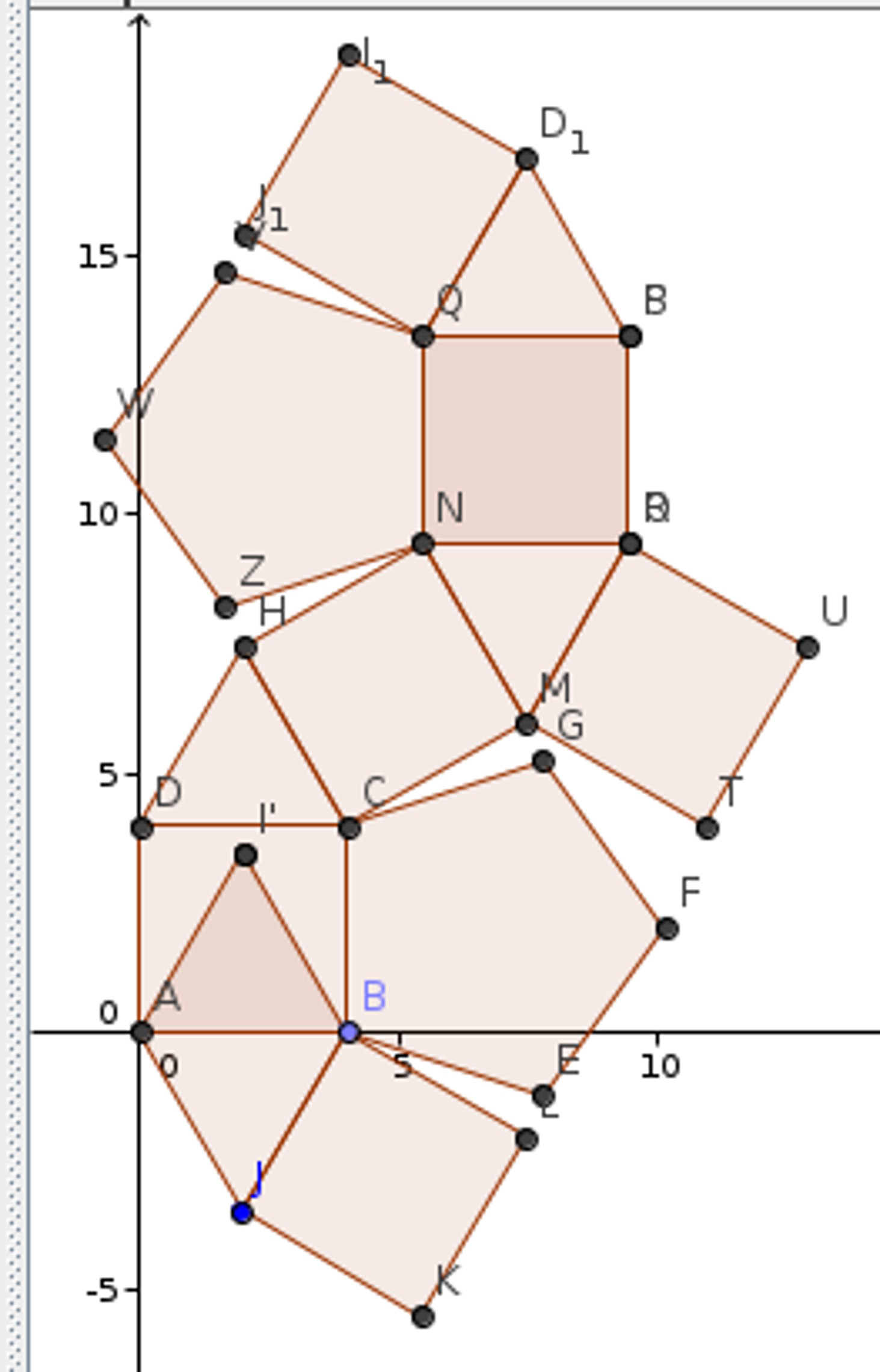# 543 models

• Posted on: 15 November 2015
• By: ashok
Tags:

What I call a 543 model is correctly called a rhombicosidodecahedron. It is often abbreviated as RID.

As prior to thinking about this I had taught the students of Vigyan Ashram how to build a big lantern based on only squares and equilateral triangles, it was logical to call this shape based on pentagons, squares and equilateral triangles as 543 reflecting the number of sides of the shapes inside it. The previous shape is then called 43. Here are two pictures.RhombicosidodecahedronRhombicosidodecahedron

The object does not have a simple symmetry. It has a five fold symmetry (almost). Here is a net of the object. Note the extra portion in the right arm portion.Rhombicosidodecahedron net

Here is the net that I used. It is one fifth but leaves two holes at the top and bottom. It is made in GeoGebra, a geometry software package providing for both graphical and algebraic input.Rhombicosidodecahedron net